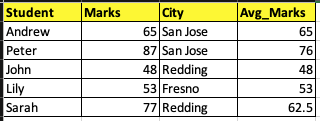top of pageSearch

# Data Through SQL Windows

Today's world contains so much data in the applications that we need advanced SQL functions to analyze this data. Few of these advanced analytics functions are are grouped under Window Functions for SQL.

Lets analyze the different window functions available in SQL

Consider a dataset of students named Report with there marks and city where they live1. PARTITION BY

To find average marks of students from a particular city we can write the query as below

select student, marks, city, avg(marks) over (partition by city order by student) from report order by student;2. PRECEDING AND FOLLOWING

To find the marks and the minimum marks of each student and the students just before and after them we can write the below query

select

student, marks,

min(marks) over (order by student ROWS between 1 preceding and 1 following)

from report order by student;3. ROW_NUMBER, RANK and DENSE_RANK

To find a unique number for each row even if they are equal we can use the row_number function.

To find the rank or dense_rank is the difference is if two values are equal then rank will skip one number and dense_rank will give the next number.

select student, marks,

row_number() over (order by marks),

rank() over (order by marks),

dense_rank() over (order by marks)

from report order by marks;To compare data of current row with previous and next row we can use lead and lag functions.

Consider a class report where we wanted to see the marks of the student in front of us and the number of marks we got more than the student behind us by

select student, marks,

lag(marks, 1) over (order by marks) as marks_of_student_infront_of_me,